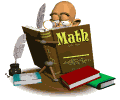• Course Information
Mathematics I will deepen and extend understanding of linear relationships, in part by contrasting them with exponential phenomenon, and in part by applying linear models to data that exhibit a linear trend. Students will use properties and theorems involving congruent figures to deepen and extend understanding of geometric knowledge. Algebraic and geometric ideas are tied together. Students will experience mathematics as a coherent, useful, and logical subject that makes use of their ability to make sense of problem situations.Syllabus Homework Study Your Notes Each Night! Supply List See Syllabus! Grading Scale See Syllabus!